# Privalov theorem

Privalov's theorem on conjugate functions: Let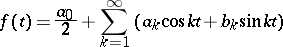be a continuous periodic function of periodand let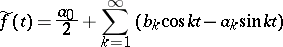be the function trigonometrically conjugate to(cf. also Conjugate function). Then ifsatisfies a Lipschitz condition of order,,, then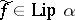forand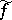has modulus of continuity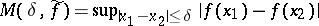at mostfor. This theorem, proved by I.I. Privalov , has important applications in the theory of trigonometric series. It can be transferred to Lipschitz conditions in certain other metrics (cf. e.g. ).

Privalov's uniqueness theorem for analytic functions: Letbe a single-valued analytic function in a domainof the complex-plane bounded by a rectifiable Jordan curve. If on some setof positive Lebesgue measure on,has non-tangential boundary values (cf. Angular boundary value) zero, thenin. This theorem was proved by Privalov ; the Luzin–Privalov theorem (cf. Luzin–Privalov theorems) is a generalization of it. See also Uniqueness properties of analytic functions.

Privalov's theorem on the singular Cauchy integral, or Privalov's main lemma, is one of the basic results in the theory of integrals of Cauchy–Stieltjes type (cf. Cauchy integral). Let: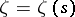,, be a rectifiable (closed) Jordan curve in the complex-plane; letbe the length of; letbe the arc length onreckoned from some fixed point; letbe the angle between the positive direction of the-axis and the tangent to; and letbe a complex-valued function of bounded variation on. Let a point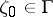be defined by a value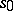of the arc length,,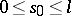, and let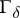be the part ofthat remains when the shorter arc with end-pointsand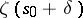is removed from. The limit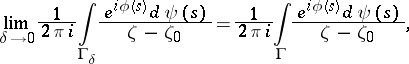(1)

if it exists and is finite, is called a Cauchy–Stieltjes singular integral. Let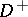(respectively,) be the finite (infinite) domain bounded by. A statement of Privalov's theorem is: If for almost-all points of, with respect to the Lebesgue measure on, the singular integral (1) exists, then almost-everywhere onthe non-tangential boundary values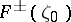of the integral of Cauchy–Stieltjes type,(2)

exist, taken respectively fromor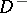, and almost-everywhere the Sokhotskii formulas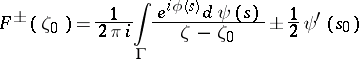(3)

hold. Conversely, if almost-everywhere onthe non-tangential boundary value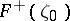(or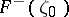) of the integral (2) exists, then almost-everywhere onthe singular integral (1) and the boundary value from the other side,(respectively,) exist and relation (3) holds. This theorem was established by Privalov for integrals of Cauchy–Lebesgue type (i.e. in the case of an absolutely-continuous function, cf. ), and later in the general case . It plays a basic role in the theory of singular integral equations and discontinuous boundary problems of analytic function theory (cf. ).

Privalov's theorem on boundary values of integrals of Cauchy–Lebesgue type: If a Jordan curveis piecewise smooth and without cusps and if a complex-valued function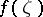,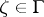, satisfies a Lipschitz conditionthen the integral of Cauchy–Lebesgue type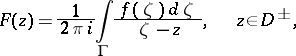is a continuous function in the closed domains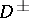. Moreover, the boundary values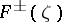satisfy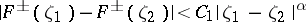for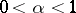, and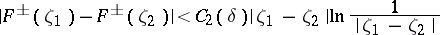for,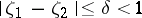(cf. ).